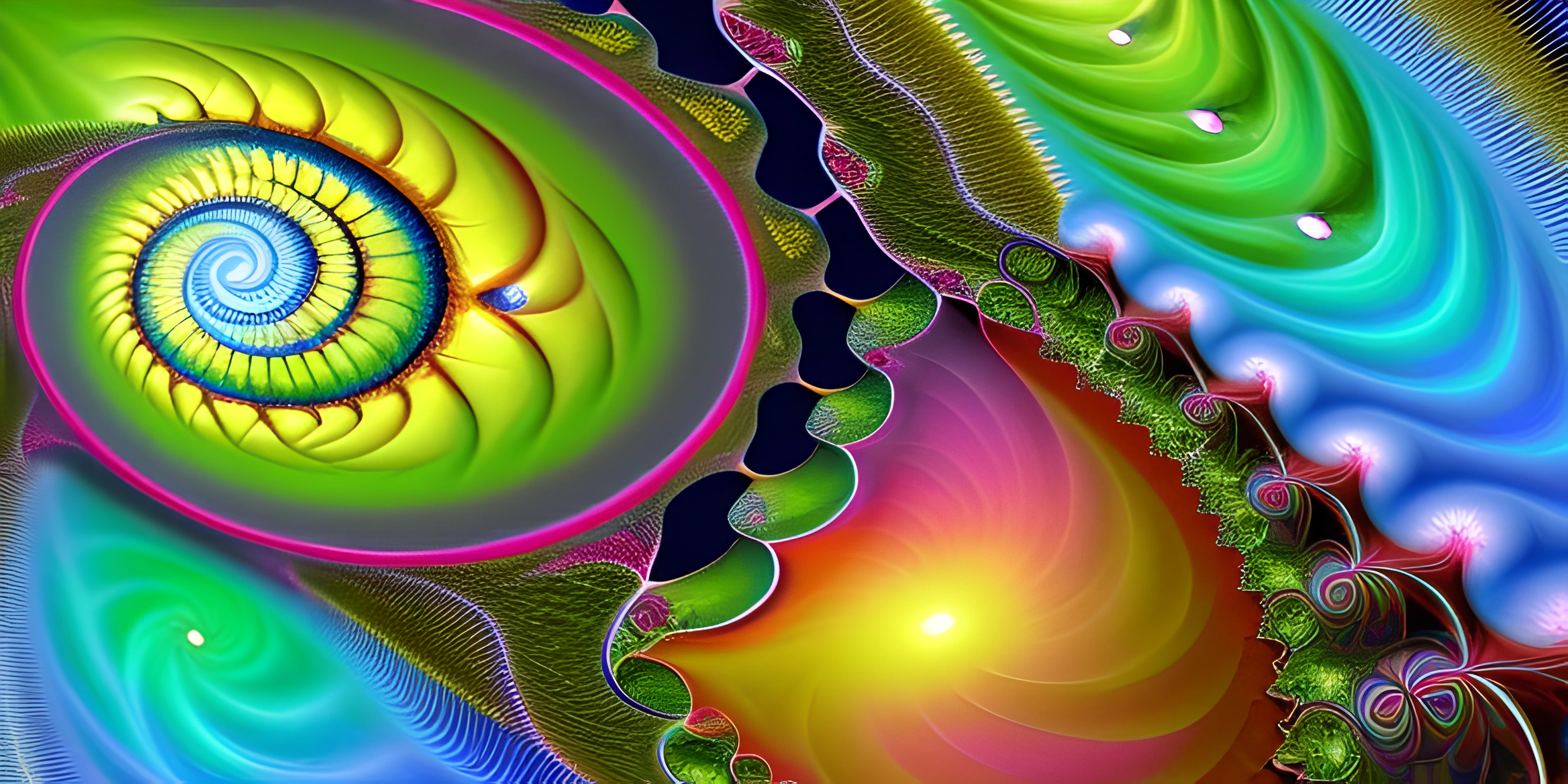# Fractal Art IntroductionNote: this page has been created with the use of AI. Please take caution, and note that the content of this page does not necessarily reflect the opinion of Cratecode.

Fractal art, a mesmerizing form of visual expression, is a delightful intersection of mathematics, programming, and aesthetics. It's like nature's doodle pad, where intricate patterns emerge from seemingly simple rules. If you've ever been captivated by the hypnotic beauty of a coastline or the never-ending complexity of a fern leaf, then you've already experienced the magic of fractals.

## What are Fractals?

Fractals are complex, infinitely detailed patterns that are self-similar across different scales. They can be found in nature, such as in the branching patterns of trees, the spiraling petals of a flower, or the jagged coastline of an island. In fractal art, these fascinating patterns are created through mathematical formulas and algorithms.

## The Connection with Generative Art

Fractal art is a subset of generative art, a broader category of art that is created with the use of algorithms or rules-based systems. Generative art embraces unpredictability and embraces the beauty of randomness. In the case of fractal art, the generative process is based on the recursive application of mathematical formulas.

## Creating Fractal Art with Programming

To create fractal art, artists use programming languages and specialized software that can handle the complex calculations needed to generate these intricate patterns. Some popular programming languages used for creating fractal art include Python, C++, and Processing.

With a programming language and a mathematical formula in hand, artists can create fascinating fractal images by iteratively applying the formula to a set of initial conditions. This recursive process generates intricate, self-similar patterns, resulting in the stunning visual complexity that characterizes fractal art.

Here's a simple example of a fractal algorithm using Python and the Turtle graphics library:

``````import turtle

def draw_fractal(length, depth):
if depth == 0:
turtle.forward(length)
else:
draw_fractal(length / 3, depth - 1)
turtle.left(60)
draw_fractal(length / 3, depth - 1)
turtle.right(120)
draw_fractal(length / 3, depth - 1)
turtle.left(60)
draw_fractal(length / 3, depth - 1)

turtle.speed(0)
draw_fractal(300, 4)
turtle.done()``````

This code generates a simple fractal pattern called the Koch curve.

## Conclusion

Fractal art is a fascinating realm where mathematics, programming, and aesthetics come together to create mesmerizing, infinitely intricate patterns. As a form of generative art, it showcases the power of algorithms and the beauty of randomness in the creative process. Dive into the world of fractal art and unleash your creativity through the endless possibilities of mathematical patterns and programming.

## FAQ

### What is fractal art and how is it related to generative art?

Fractal art is a form of digital art that utilizes mathematical algorithms to create visually complex and fascinating patterns or images. It is derived from fractals, which are geometric shapes that can be split into smaller parts, each of which is a reduced-scale copy of the whole. Fractal art is closely related to generative art, as both involve the use of algorithms and programming to generate unique and intricate visuals.

### How are fractals used in creating art?

Fractals are used in creating art by employing mathematical algorithms that define and manipulate the shapes and patterns within the design. By adjusting the parameters of the algorithm, artists can create an infinite variety of fractal images, each with its own unique characteristics. These images often exhibit self-similarity and intricate detail, which make them visually appealing and engaging.

### Can I create my own fractal art without prior programming knowledge?

Yes, you can create your own fractal art even if you don't have prior programming experience. There are several user-friendly software programs and tools available that allow you to generate fractal images by simply adjusting parameters and exploring various settings. Some popular options include Mandelbrot Set viewers, Apophysis, and Ultra Fractal.

### How is mathematics involved in the creation of fractal art?

Mathematics plays a crucial role in the creation of fractal art, as it provides the foundation for the algorithms that generate the intricate patterns and shapes seen in the images. Fractal art relies on mathematical concepts such as iteration, recursion, and complex numbers to create visually stunning and infinitely complex designs. The beauty of fractal art lies in the way it visually represents complex mathematical concepts in an accessible and engaging manner.

### Can you provide an example of a popular fractal algorithm used in art?

One popular fractal algorithm used in creating art is the Mandelbrot Set. Named after the mathematician Benoit Mandelbrot, this algorithm generates a set of complex numbers that, when plotted on a graph, create an intricate and mesmerizing pattern. The Mandelbrot Set is defined by the iterative formula: Z_n+1 = Z_n^2 + C where Z_n is a complex number, C is a constant, and n represents the iteration number. By plotting the points that remain bounded in the complex plane, the resulting image reveals the captivating and intricate designs characteristic of fractal art.